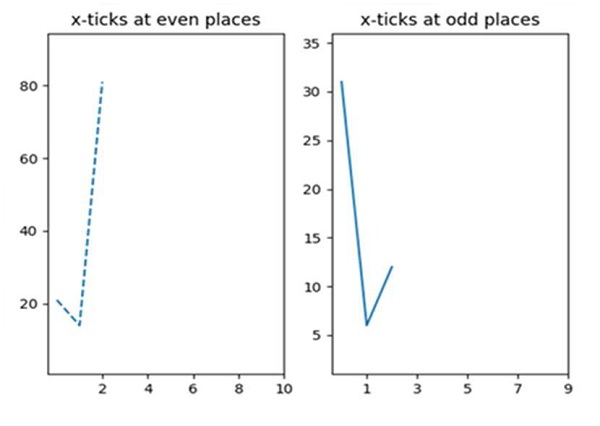# Python xticks in subplots

PythonMatplotlibServer Side ProgrammingProgramming

#### Beyond Basic Programming - Intermediate Python

Most Popular

36 Lectures 3 hours

#### Practical Machine Learning using Python

Best Seller

91 Lectures 23.5 hours

#### Practical Data Science using Python

22 Lectures 6 hours

Subplot can split the figure in nrow*ncols parts and plt.xticks could help to plot the xticks for subplots.

## Steps

• Create two lists for line 1 and line 2.

• Add a subplot to the current figure, nrow = 1, ncols = 2 and index = 1.

• Draw line 1 with style as dashed.

• Set or retrieve auto scaling margins(0.2).

• Place xticks at even places.

• Set a title for the X-axis.

• Add a subplot to the current figure, nrow = 1, ncols = 2 and index = 2.

• Plot line 2.

• Set or retrieve auto scaling margins(0.2).

• Place xticks at odd places.

• Set a title for the X-axis.

• To show the figure use plt.show() method.

## Example

import matplotlib.pyplot as plt

line1 = [21, 14, 81]
line2 = [31, 6, 12]

plt.subplot(121)
plt.plot(line1, linestyle='dashed')
plt.margins(0.2)
plt.xticks([2, 4, 6, 8, 10])
plt.title("x-ticks at even places")

plt.subplot(122)
plt.plot(line2)
plt.margins(0.2)
plt.xticks([1, 3, 5, 7, 9])
plt.title("x-ticks at odd places")

plt.show()

## Output